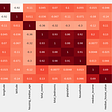# Introduction

In the advent of gradient boosted (GB) decision trees (adaBoost, XGBoost, LGBM), such systems have gained notable popularity over other tree-based methods such as Random Forest (RF). Though both have their place in the data science roadmap, in my experience I tend to favour GB models over RF due to the incredibly fast training speed.

Though as the title might suggest, I have a problem with GB models, one which isn’t typically made clear to Data Scientists. There is a gremlin in the gradient boosting algorithm, one that, if not accounted for, may break a data science pipeline when it’s too late.

# The Problem

First, let’s frame the problem with some code! now as a pre-warning, this article is to explain at a top-level how these algorithms work and where to be careful in their implementation. It is NOT a tutorial on how to effectively use these models, so get ready for some bad data science choices.

Now, what is the problem you might ask? well, the standard assumption I make (and I’m sure a lot of other data scientists make) when using tree-based regressors is:

A tree-based regressor will produce predictions in the range in which they’re trained on

Meaning if we have some training dataset with target `y_t` and features `X_t`, where `y_t` has a range `[a, b]`. Once we’ve fit a model to our dataset, we expect all our predictions `y_p`, from a new set of features `X_p`, regardless of what new values `X_p` contains, to be in the range `[a, b]`. BUT you see, unlike decision trees or RF algorithms, this isn’t the case with GBT models, GBT models can predict outside the range `[a, b]`!!

Now you might ask why this is an issue?

Well, here at Gousto DS, we were in the stages of releasing a new model for predicting the relative popularity of recipes for a given week that used LGBM. This problem space requires all predictions to be a positive float, as relative popularity can only be greater than 0.

You might think we’re safe using a tree-based regressor because if you only train on positive values, it can never be negative! right? Well fortunately before our new recipe popularity model hit production we saw something weird; a gradient boosted gremlin kicked our popularity predictions outside the training range and made a negative prediction!

Let’s demonstrate this happening with some public dataset, first let’s import some libraries and get some data:

Now, select some arbitrary features to train on `X_train` and fit our models to `y_train`, here we’re just predicting the distance of a taxi journey, ie: must always be a positive float.

Now let’s look at what our target feature looks like:

So our range for `y_train` in our training dataset is `[0, 36.7]`.

Now let’s create some models! for this example I’m going to compare:

• An RF model, the sklearn `RandomForestRegressor`
• My personal favourite GBT model, `lightgbm`

For fitting the random forest we run:

For our `lightgbm` model, it’s a bit different, we define most of our model parameters in a dictionary, create a `Dataset` class, then just pass the parameters and `Dataset` at the training stage to the `lightgbm` class:

GREAT! now we have two models fitted to our dataset, `regr` and `lgbmodel` .

What we want to do next is create some toy data to predict on. It doesn’t matter if the data makes sense, we just want a lot of data with a variety of feature values `X_predict` to predict from.

If we plot our predictions `y_predict`, on top of our training values `y_train`, we can see how GB models can predict outside our `y_train` range of `[0, 36.7]`! whereas the RF model does what we expect and keeps inside the range.

# The Theory

Okay, so I’ve proven to you that gremlins exist, but now you might ask, why does this happen?

Well before we dive into why this happens, let’s just refresh our memory on how tree-based methods work (for more reading on this see Chapter 8 of An Introduction to Statistical Learning).

## Decision Trees

Before even training/fitting a model to our data, we need to define a loss function, a standard one is Mean Squared Error (MSE). This is simply:

• Given `i` observations with feature set `X`, and true values `y_hat`
• When we train a model M
• Then our model makes `i` predictions `y`

The loss is the mean of the squared differences between the predictions `y` and the true values `y_hat`:

The aim of training is to minimise MSE when growing the tree, we can evaluate MSE at each node that we split.

To grow a tree, we then recursively split the data into `J` regions `R_j` across our feature space `X`. The prediction value assigned to the region `R_j` is the value that minimises the MSE Loss function. This is visualised below for a tree that has grown into 5 regions across some 2D feature space `X`.

This kind of predictor can’t make predictions outside of the range `[a, b]` the model was trained on. This is because, for any new observation with features `X_p` that are outside the training range of our feature set `X_t`, the prediction value for `X_p`, `y_hat`, will just fall on a flat plane. aka. a region `R`, which is a plane perpendicular to `y` and within the bounds `[a, b]` (see figure above).

We can generalise our model f(X) as: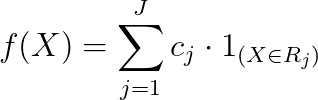Where R_1 ….. R_J represents a partition of feature space, and c_j, represents the value adjustment for the jth feature space partition.

## Random Forests

For random forests, we grow multiple trees B, each trained on a bootstrapped dataset, and grown on a random sample of features at each split. (for more reading on this see Chapter 8 of An Introduction to Statistical Learning).

When making a prediction, we get each tree from our set of trees B, to each make a prediction, we then average the results to get our final prediction `y_t` (like all good data science algorithms, just average it). Now each tree will be bound to the same logic we just defined:

the prediction value, y_hat, will just fall on a flat plane, ie: a region R, which is within the bounds [a,b]

The only difference is, we are averaging results across many trees, but an average of results within bounds `[a, b]` will still be a result within bounds `[a, b]`.

We can generalise our model for RF as: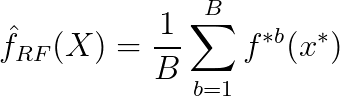Where f*b(x*) is the tree grown for the bth bootstrapped dataset on the x* randomly sampled features.

For GB methods, it gets a bit more complicated, so grab a coffee and get ready to join me on this rabbit hole.

In GB models, we grow our trees in a similar way to how we grow our decision tree above (albeit there are numerous differences in reality, ie: pre-sort-based algorithms, histogram-based algorithms, level vs leaf-wise growth etc, see the original LightGBM paper for more details on these).

The main difference between RF and GB models is that where RF takes the average of many models, GB trees are additive, meaning we sum the predictions between all trees. The way the GB model f_GBT(X) fits to a dataset (X, y) is:

1. Set f_GBT(X) = 0, giving residuals `r_i = y_i` for all i data points.
2. For b = 1, 2, …. B trees, repeat:

a) Fit tree f^b with d splits to the training data (X, r)

b) Update f_GBT(X) by adding in a shrunken version of the new tree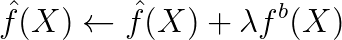where lambda is the learning rate or shrinkage parameter typically in the range {0, 1}

c) Update the residuals that will be used for the next tree

This gives our boosted model in the form:

The main takeaway from this is that (in point (a) above) we fit our tree f^b to the residuals of our model at that boosting round! aka. the residuals of each tree we grow do not start at `r_i = y_i`

In gradient boosted trees, new trees are created to correct the residual errors in the predictions from the existing sequence of trees

Though it isn’t glaringly obvious that this is the reason why we can make predictions outside our target range `[a, b]`, I’ll do my best to demonstrate this. But before this, let’s just briefly touch on how GBT methods calculate loss.

Now, with this additive model in mind, and knowing that when we grow our trees, we need to keep track of our loss function, something like MSE loss becomes a bit more complicated and computationally expensive from this kind of additive model.

This is surprisingly one of the simpler loss function derivations for GB models! To make loss functions simpler for GB models, we take a 2nd order Taylor series expansion of our loss function to approximate it at a given boosting round in our model training phase, (note this is a bit of a pain if you ever want to use linear loss functions as there’s no 2nd order Tayor series of a linear function).

Doing this makes our loss function far less computationally expensive as our first order Taylor term, `g_i` (gradient) and second-order term `h_i` (hessian) become constant for each boosting round. This is one of the main benefits of GB models.

Gradient boosted trees are used to minimise an approximate loss function, not to respect the bounds of initial inputs. It sees only the gradient statistics, which is the couple (gradient, hessian) for each trained observation.

Now that we have a bit of a better understanding of the inner mechanics of GBT models, let’s smoke out the gremlin!

## Gradient Boosted Trees (smoking out the gremlin)

The primary cause to predicting outside a training range is due to the additive nature of gradient boosted models.

Now, to find these gremlins, I’m going to do two things to demonstrate how using an additive model can create a gremlin:

• Use a very simple training dataset so we don’t get lost in numbers
• Burn and cut down most of the GB forest (metaphorically of course)

For my model, I’m only going to:

• grow stumps (max_depth = 1)
• increase the learning rate to 1 to get rid of that troublesome lambda/shrinkage parameter
• Grow only 2 stumps (boosting_rounds = 2).

This should help me smoke out those gremlins. With these parameters, my fitted model is:

So simple that we can calculate the results with pandas:

Giving:

Here we can see how our residuals `r_i` get reduced at each step. The end predictions are relatively sensible from the albeit, terrible training data. There’s a super interesting point to make here, see how for row index 2 we:

• have `y = 0.5` and a residual `r_0 = 0.5`.
• Our first fitted stump makes it so our prediction is `f_1(X) = 0.75`, now our residual is `r_1 = 0.5 – 0.75 = -0.25`
• when fitting our second stump, our model sees `r_1 = -0.25` and says “hey! I can reduce this residual to 0! I just need to make a split so that if `feature_1 > 0` I add 0.25”
• And we get a `y_pred` that equals our true `y` value, 0.5

This seems fine, but what happens if we add an extra row with `feature_0 = 0` and `feature_1 = 1` ?

We got a prediction of -0.25! which is outside our training range of [0, 1]! And thus, the additive nature of our model has created a gremlin capable of kicking our predictions outside our training range.

# The Solution

Okay, so we understand how these gremlins work, but now you might ask, what can we do about it?

My favourite suggestion that I’ve come across so far is from this closed Github issue for XGBoost, where we simply bound all negative samples to the minimum of our initial predictions. This is what we decided to do for our recipe popularity predictor.

Another suggestion is to create a large loss on negative samples in a custom loss function, though this might be harder to implement.

Though personally, I’m not satisfied with these solutions as they feel like band-aids. If there’s a better solution out there, I’ll be sure to write about it soon.

--

--

--

## More from Gousto Engineering & Data

Gousto Engineering & Data Blog

## Prediction Employee Turnover: a machine learning approach.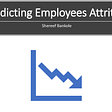## Breast Cancer Detection Using Machine Learning## Raven Protocol AMA with Sherman Lee in the Binance AMA Series## Linear Regression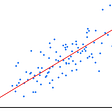## Fast.ai Lesson 5 notes — Part 1 v3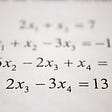## Understanding RMSprop — faster neural network learning## K-means clustering for faster collaborative filtering## Can my computer understand me?## More from Medium## Feature selection using FRUFS and VevestaX## Latest Interview Data Science Questions My Friends and I got asked in 2022## Hypothesis Testing and Confidence Intervals using Python